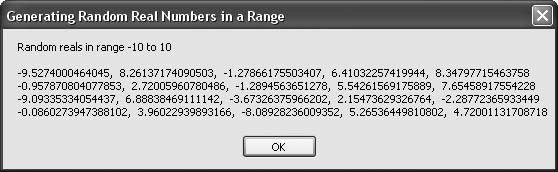# Recipe 6.28. Generating Random Real Numbers in a Range

#### Problem

You need to generate a sequence of pseudorandom real numbers with a flat distribution over a given range.

#### Solution

Sample code folder: Chapter 06\RepeatRandom

The BetterRandom class (see Recipe 6.26) sports a GetNextreal() function. Two parameters define the range limits for the returned pseudorandom real values, and the returned value has a statistically flat distribution across the given range:

` GetNextReal(minReal, maxReal) `

#### Discussion

The following code creates a new instance of the BetterRandom object, which it then uses to generate 20 pseudorandom double-precision real numbers in the range -10.0 to +10.0. The results are collected and then displayed for review. As a programming exercise, you might consider changing this code to display the average and perhaps the standard deviation for these returned values.

The generator object is created without passing a string to initialize the generator, so a unique sequence will be created every time this program is run:

` Dim result As New System.Text.StringBuilder Dim generator As New BetterRandom Dim minReal As Integer = -10 Dim maxReal As Integer = 10 Dim counter As Integer result.Append("Random reals in range ") result.AppendLine(minReal & " to " & maxReal) result.AppendLine() For counter = 1 To 20    ' ----- Add one random number.    result.Append(generator.GetNextReal(minReal, maxReal))    If ((counter Mod 5) = 0) Then       ' ----- Group on distinct lines periodically.       result.AppendLine()   Else      result.Append(", ")   End If Next counter MsgBox(result.ToString()) `

Figure 6-28 shows the results of generating the 20 pseudorandom double-precision real values.

##### Figure 6-28. Pseudorandom reals in the range -10.0 to +10.0 generated by the BetterRandom objectRecipe 6.26 shows the full code for the BetterRandom class.

There are many good references on the Web to learn more about random number generation (see, for example, http://random.mat.sbg.ac.at).Visual Basic 2005 Cookbook: Solutions for VB 2005 Programmers (Cookbooks (OReilly))
ISBN: 0596101775
EAN: 2147483647
Year: 2006
Pages: 400

Similar book on Amazon